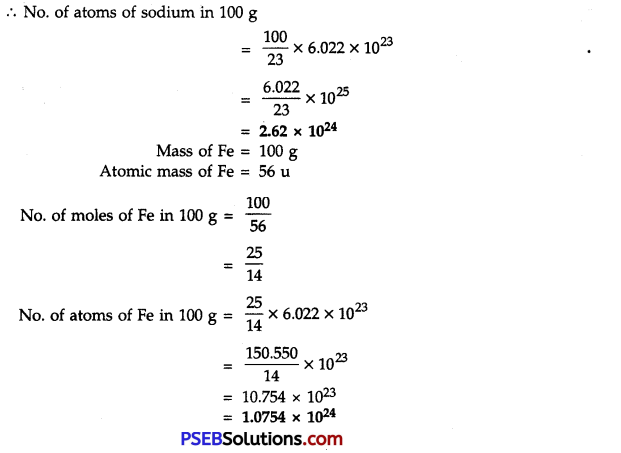# PSEB 9th Class Science Solutions Chapter 3 Atoms and Molecules

Punjab State Board PSEB 9th Class Science Book Solutions Chapter 3 Atoms and Molecules Textbook Exercise Questions and Answers.

## PSEB Solutions for Class 9 Science Chapter 3 Atoms and Molecules

PSEB 9th Class Science Guide Atoms and Molecules Textbook Questions and Answers

Question 1.
A 0.24 g sample of a compound of oxygen and boron was found by analysis to contain 0.096 g of boron and 0.144 g of oxygen. Calculate the percentage composition Of the compound by weight.
Weight of compound = 0.24 g
Weight of Boron = 0.096 g
Weight of oxygen = 0.144 g
∴ % age of B = $$\frac{0.096}{0.24}$$ × 100
= $$\frac{96}{240}$$ × 100 = 40
% age of O = $$\frac{0.144}{0.240}$$ × 100 = 60Question 2.
When 3.0 g of carbon is burnt in 8.00 g oxygen, 11.00 g of carbon dioxide is produced. What mass of carbon dioxide will be formed when 3.00 g of carbon is burnt in 50.00 g of oxygen? Which law of chemical combination will govern your answer?
3.00 g of carbon combines with 8.00 g of oxygen to produce 11.00 g of carbon dioxide
C + O2 → CO2
When 3.00 g of carbon is burnt in 50.00 g of oxygen, 11.00 g of carbon dioxide is produced. This is based upon the law of constant compositions.

Question 3.
What are polyatomic ions? Give examples.
Polyatomic ions. A group of atoms carrying a charge (+ve or -ve) is called a polyatomic ion.
Examples: $$\mathrm{SO}_{4}^{2-}$$, $$\mathrm{SO}_{3}^{2-}$$, $$\mathrm{NH}_{4}^{+}$$ etc.

Question 4.
Write the chemical formulae of the following :
(a) Magnesium chloride
(b) Calcium oxide
(c) Copper nitrate
(d) Aluminium chloride
(e) Calcium carbonate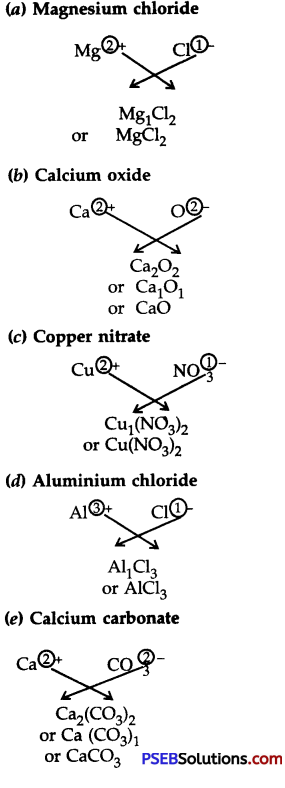Question 5.
Give the names of the elements present in the following compounds:
(a) Quick lime
(b) Hydrogen bromide
(c) Baking powder
(d) Potassium sulphate
(a) Quick lime (CaO). The elements present in it are calcium and oxygen.
(b) Hydrogen bromide (HBr). The elements present in it are hydrogen and bromine.
(c) Baking powder (NaHC03). The elements present in it are sodium, hydrogen, carbon and oxygen.
(d) Potassium sulphate (K2S04). The elements present in it are Potassium, Sulphur and Oxygen.

Question 6.
Calculate the molar mass of the following substances:
(a) Ethyne, C2H2
(b) Sulphur molecule, S8
(c) Phosphorus molecule, P4 (Atomic mass of phosphorus is 31)
(d) Hydrochloric acid, HCl
(e) Nitric acid, HNO3
(a) Molar mass of ethyne, C2H2 = 2 × 12 + 2 × 1 = 24 + 2 = 26 g
(b) Molar mass of sulphur molecule, S8 = 8 × 32 = 256 g
(c) Molar mass of Phosphorus molecule, P4 = 4 × 31 = 124 g
(d) Molar mass of hydrochloric acid, HCl = 1 + 35.5 g = 36.5 g
(e) Molar mass of nitric acid, HNO3 = 1 + 14 + 3 × 16 = 1 + 14 + 48 = 63g

Question 7.
What is the mass of:
(a) 1 mole of nitrogen atoms?
(b) 4 moles of aluminium atoms (Atomic mass of aluminium = 27)?
(c) 10 moles of sodium sulphite (Na2S03)?
(a) Mass of 1 mole of Nitrogen atoms = 14 g
(b) Mass of 1 mole of A1 atoms = 27 g
Mass of 4 moles of A1 atoms = 4 × 27 = 108 g
(c) Mass of 1 mole of sodium sulphite, Na2SO3
= 2 × 23 + 32 + 3 × 16
= 46 + 32 + 48 = 126 g
∴ Mass of 10 moles of Na2SO3 = 10 × 126 = 1260 gQuestion 8.
Convert into moles:
(a) 12 g of oxygen gas
(b) 20 g of water
(c) 22 g of carbon dioxide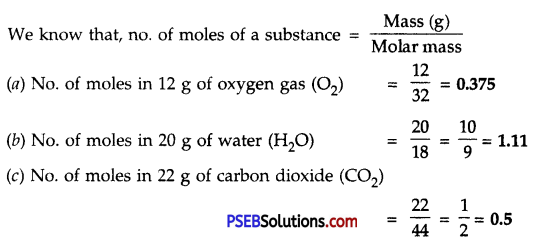Question 9.
What is the mass of:
(a) 0.2 mole of oxygen atoms?
(b) 0.5 mole of water molecules?
(a) 0.2 mole of oxygen atoms:
Mass of 1 mole of oxygen atoms = 16 g
Mass of 0.2 mole of oxygen atoms = 0.2 × 16 = 3.2 g
(b) 0.5 mole of water molecules:
Mass of 1 mole of water molecule (H2O) = 18 g
∴ Mass of 0.5 mole of water molecules = 0.5 × 18 = 9 g

Question 10.
Calculate the number of molecules of sulphur(S8) present in 16 g of solid sulphur.
Mass of solid sulphur (S8) = 16 g
Mass of 1 mole of sulphur (S8) = 8 × 32 = 256 g
No. of molecules in 16 g of solid sulphur = 16/256 × 6. 023 × 1023
= 3.76 × 1022 moleculesQuestion 11.
Calculate the number of aluminium ions present in 0.051 g of aluminium oxide.
(Hint: The mass of an ion is the same as that of an atom of the same element.
The atomic mass of Al = 27 u)
Mass of 1 mole of aluminium oxide (Al2O3) = 2 × 27 + 3 × 16 = 54 + 48 = 102 g
1 mole of Al2O3 contains Al3+ ions = 2 × 27 = 54 g
or 102 g of Al2O3 contains Al3+ contains = 54 g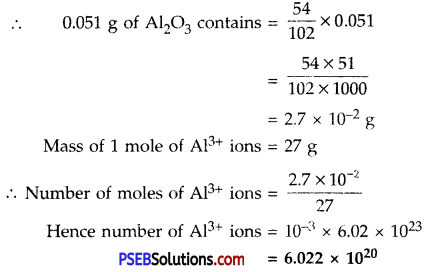Science Guide for Class 9 PSEB Atoms and Molecules InText Questions and Answers

Question 1.
In a reaction, 5.3 g of sodium carbonate reacted with 6 g of ethanoic acid. The products were 2.2 g of carbon dioxide, 0.9 g water and 8.2 g of sodium ethanoate. Show that these observations are in agreement with the law of conservation of mass.
Sodium carbonate + ethanoic acid → sodium ethanoate + carbon dioxide + water.
Sodium carbonate + ethanoic acid → sodium ethanoate + carbon dioxide + water.

• Mass of sodium carbonate = 5.3 g
• Mass of ethanoic acid = 6.0 g
• Total mass of the reactants = 5.3 + 6.0 = 11.3g
• Mass of carbon dioxide = 2.2 g
• Mass of water = 0.9 g
• Mass of sodium ethanoate = 8.2 g
• Total mass of the products = 2.2 + 0.9 + 8.2 = 11.3 g

Since total mass of the reactants = Total mass of the products
Hence, the Law of Conservation of Mass is true.

Question 2.
Hydrogen and oxygen combine in the ratio of 1:8 by mass to form water. What mass of oxygen gas would be required to react completely with 3 g of hydrogen?
One g of hydrogen gas reacts with oxygen = 8 g
3 g of hydrogen gas will react with oxygen = 3 × 8 = 24 gQuestion 3.
Which postulate of Dalton’s atomic theory is the result of the law of cosnervation of mass?
Law of conservation of mass is based upon the postulate that atoms are indivisible particles and can neither be created nor destroyed during any chemical reaction.

Question 4.
Which postulate of Dalton’s atomic theory can explain the law of definite proportions?
Atoms of various elements combine in simple whole-number but the fixed ratio to form compound atoms (molecules).

Question 5.
Define the atomic mass unit.
One atomic mass unit is a mass unit and it is equal to 1/12th of the mass of an atom of carbon-12.
1 a.m.u. = 1.66 × 10-27 kg

Question 6.
Why is it not possible to see an atom with naked eyes?
This is because atoms are very-very small. The radius of an atom is of the order of 10-10 m.

Question 7.
Write down the formulae of
1. Sodium oxide
2. Aluminium chloride
3. Sodium sulphide
4. Magnesium hydroxide.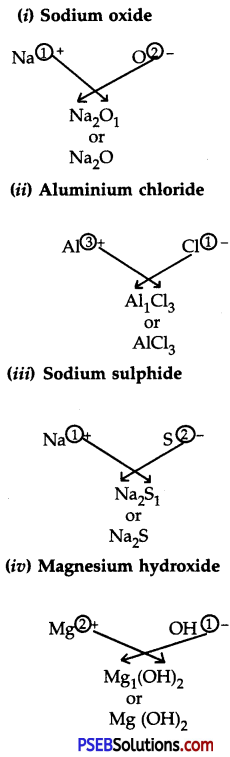Question 8.
Write down the names of the compounds represented by the following formulae,

1. Al2 (S04)3
2. CaCl2
3. K2S04
4. KN03
5. CaCO3

1. Aluminium sulphate
2. Calcium chloride
3. Potassium sulphate
4. Potassium nitrate
5. Calcium carbonate.Question 9.
What is meant by the term chemical formula?
Chemical formula. The chemical formula of a substance indicates its constituent elements and number of atoms of each combining element present in One molecule of it.

Question 10.
How many atoms are present in ;
1. H2S molecule
2. $$\mathrm{PO}_{4}^{3-}$$ ion
1. An H2S molecule represents
(a) two atoms of hydrogen and
2. A $$\mathrm{PO}_{4}^{3-}$$ ion represents
(a) one atom of P
(b) three atoms of O.

Question 11.
Calculate the molecular masses of H2, O2, Cl2, CO2, CH4, C2H6, C2H4, NH3, CH3OH.

• Molecular mass of H2 = 2 × 1 = 2u
• Molecular mass of 02 = 2 × 16 = 32 u
• Molecular mass of Cl2 = 2 × 35.5 = 71.0 u
• Molecular mass of C02 = 1 × 12 + 2 × 16 = 12 + 32 = 44 u
• Molecular mass of CH4 = 12 + 4 × 1 = 12 + 4 = 16 u
• Molecular mass of C2H6 = 2 × 12 + 6 × 1 = 24 + 6 = 30 u
• Molecular mass of C2H4 = 2 × 12 + 4 × 1 = 24 + 4 = 28 u
• Molecular mass of NH3 = 14 + 3 × 1 = 14 + 3 = 17 u
• Molecular mass of CH3OH = 12 + 3 × 1 + 16 + 1 = 12 + 3 + 16 + 1 = 32 u

Question 12.
Calculate the formula unit masses of ZnO, Na2O, K2C03.
(Given atomic masses of Zn = 65 u, Na = 23 u, C = 12u and O = 16 u)

• Formula unit mass of ZnO = 65 + 16 = 81 u
• Formula unit mass of Na2O = 2 × 23 + 16 = 46 + 16 = 62 u
• Formula unit mass of K2C03 = 2 × 39 + 12 + 3 × 16 = 78 + 12 + 48 = 138 uQuestion 13.
If one mole of carbon atoms weigh 12 gram. What is the mass (in grams) of 1 atom of carbon?
Mass of 1 atom of carbon = $$\frac{12}{6.023 × 10}$$
No. of moles of sodium in 100 g = $$\frac{100}{23}$$